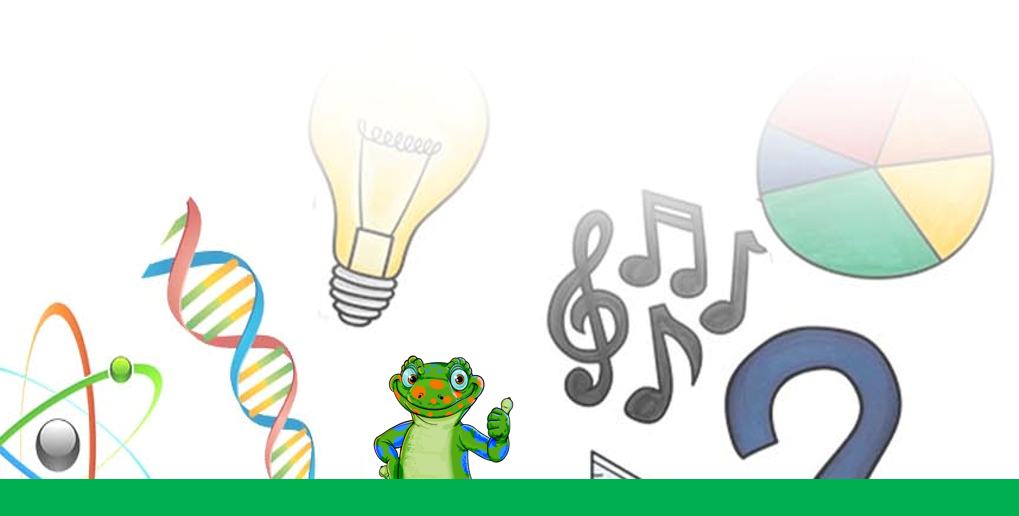### Submit a Resource

Algebra 87 listings
Mathalicious: Pandemic
Mathalicious

In this lesson, students use exponential growth and logarithms to model how a virus spreads through a population and evaluate how various factors influence the speed and scope of an outbreak.

Mathalicious: New Twenty
Mathalicious

In this lesson, students use proportions to determine what life expectancy must have been in order for 30 to really be the new 20.

Mathalicious: Carpe Donut
Mathalicious

In this lesson, students use linear, rational, and piecewise functions to describe the total and average cost of an order at Carpe Donut.

Mathalicious: I Remember
Mathalicious

In this lesson, students use exponential decay to model memory fidelity and debate whether a bad memory is a good thing.

The Kennedy Center: How Many Cells Are Born in a Day?
The Kennedy Center

In this lesson, students use calculation to predict the number of cells that result from a series of cell divisions.

Crash Course: Study Hall Algebra #4 - Understanding Fractions
Crash Course

Rational expressions (aka fractions) can be scary to see on the page.

Mathalicious: You're So Fined
Mathalicious

In this lesson, students write, solve, and graph systems of linear equations to determine how long it takes to pay off a ticket and debate the fairest ways for cities to raise revenues without harming their poorest residents.

Mathalicious: Family Plan
Mathalicious

In this lesson, students use proportional reasoning to predict whether a family will exceed their wireless plan allotment.

Mathalicious: Pyramid of Sleaza
Mathalicious

In this lesson, students learn about how pyramid schemes work, and use geometric sequences to model the (exponential!) growth of a pyramid scheme over time.

Mathalicious: House of Pain
Mathalicious

In this lesson, students use exponential decay and rational functions to understand why addicted patients seek more and stronger opioids to alleviate their pain.

The Kennedy Center: Patterns Across Cultures - The Fibonacci Sequence in Visual Art
The Kennedy Center

Exploring where the Fibonacci Sequence and Golden Ratio appear in nature and the visual arts.

Crash Course: Study Hall Algebra #3 - Negative Numbers and Arithmetic
Crash Course

In this episode of Study Hall: Algebra, James walks us through a bit of history and practical math.